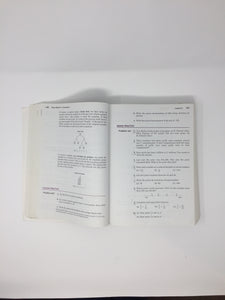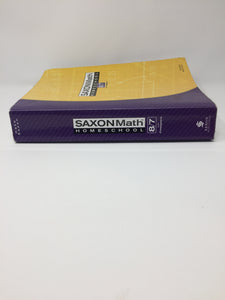# Saxon Math 8/7(Prealgebra), 3rd Edition, Student Text (Used- Good)

Regular price
Sold out
Sale price
\$24.00

Focusing on algebraic reasoning and geometric concepts, Saxon Math Homeschool 8/7 teaches math with a spiral approach, which emphasizes incremental development of new material and continuous review of previously taught concepts.

This Saxon 87 textbook reviews arithmetic calculation, measurements, geometry and other skills, and introduces pre-algebra, ratios, probability and statistics. Students will specifically learn about adding/subtracting/multiplying fractions, equivalent fractions, the metric system, repeating decimals, scientific notation, Pi, graphing inequalities, multiplying algebraic terms, the Pythagorean Theorem, the slope-intercept form of linear equations, and more .

Lessons contain a warm-up (with facts practice, mental math, & problem-solving exercises); introduction to the new concept, lesson practice exercises where the new skill is practiced, and mixed practice exercises, which includes 25-30 old and new problems. In-depth "Investigations" are provided every 10 lessons, and have their own set of questions. The back of the book contains supplemental problems for selected lessons and concepts.

931 indexed pages, softcover. 120 daily lessons, 12 investigations, and an illustrated glossary included. (cbd.com description)

Used - Good

The cover is intact but may have been written on. Spine may show signs of wear. All pages are intact but may include limited notes, highlighting, or light pencil marks. May include "From the library of" labels, stickers, or markings.